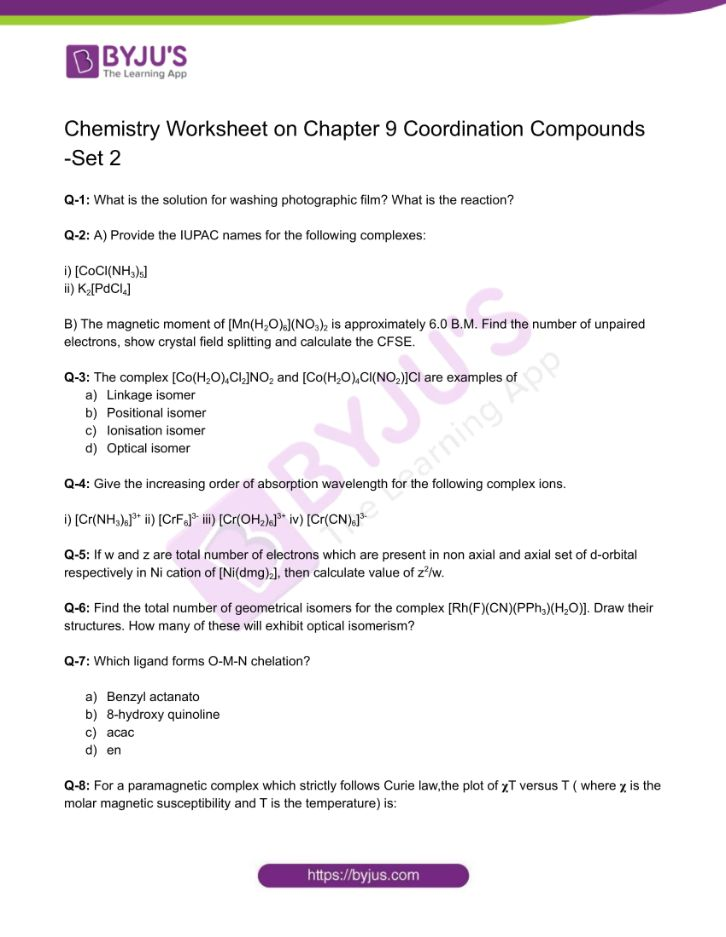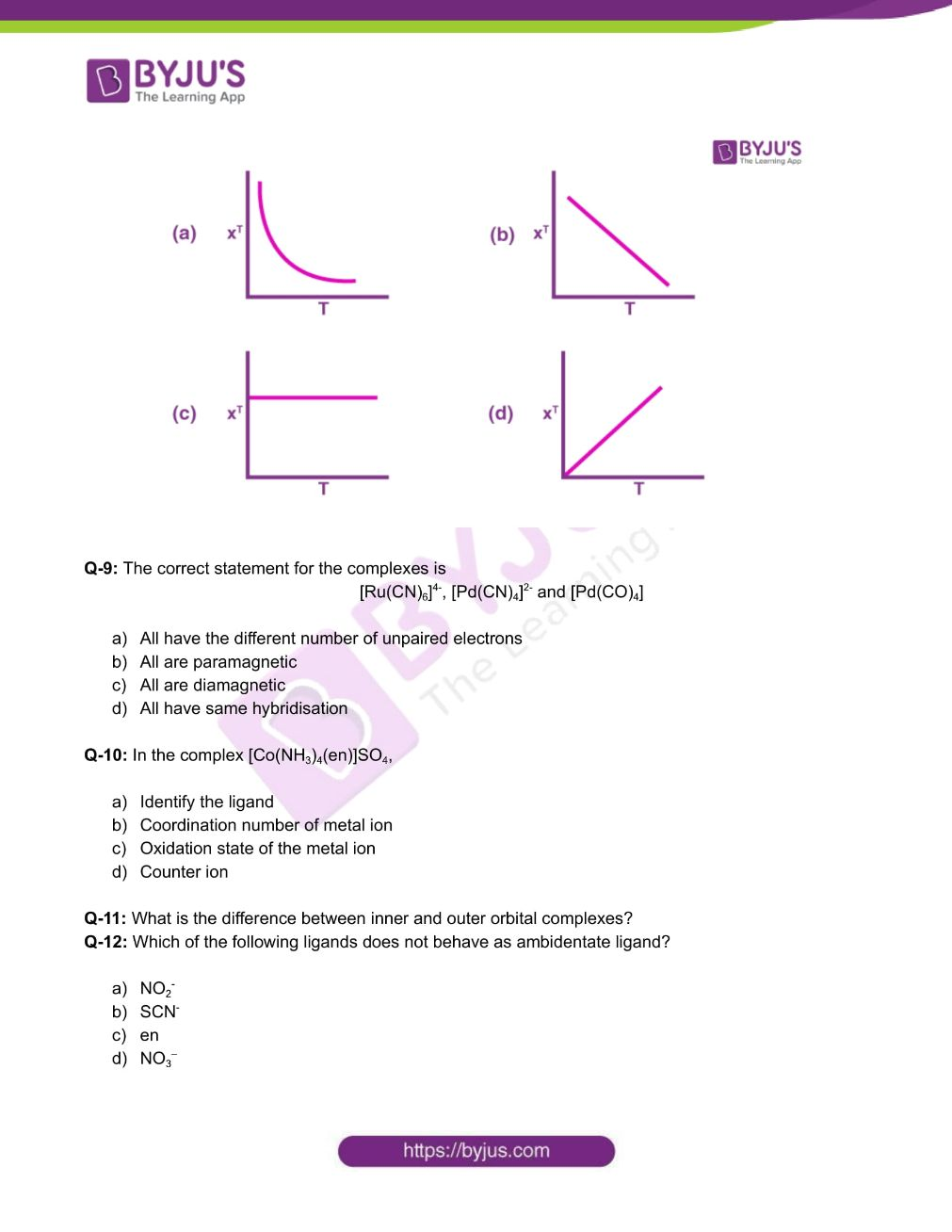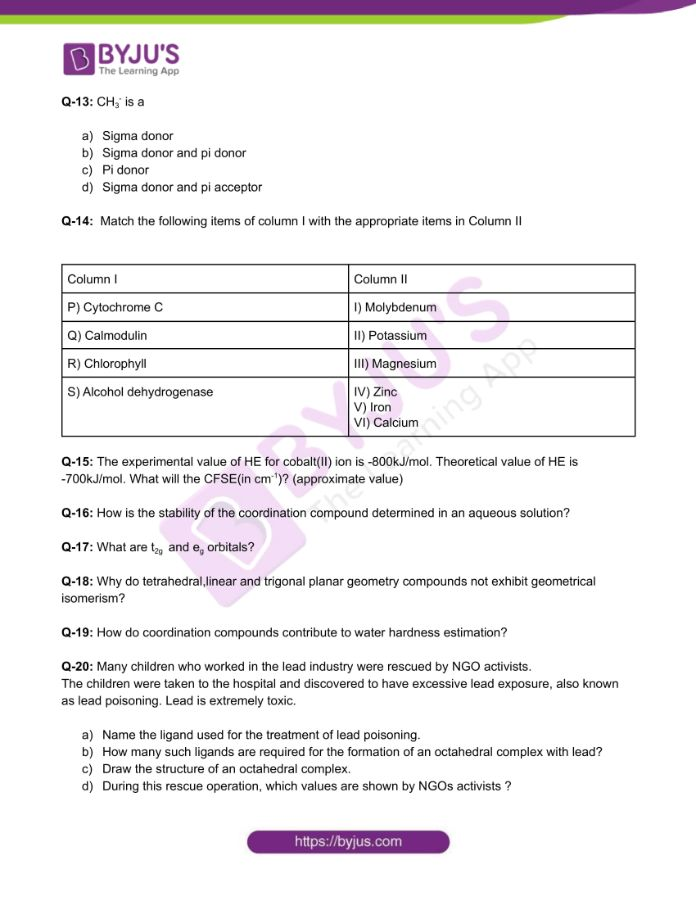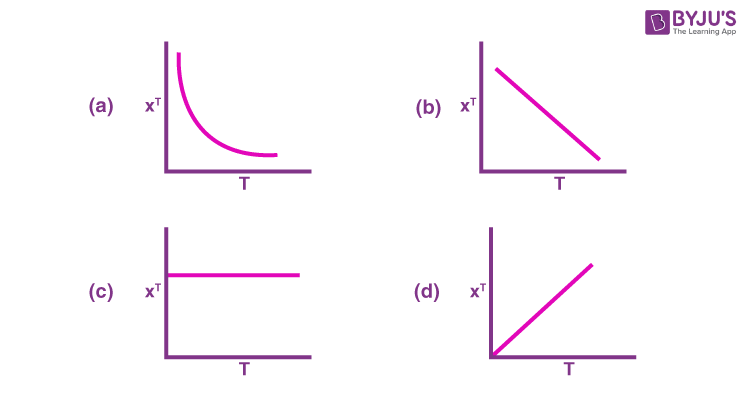Checkout JEE MAINS 2022 Question Paper Analysis : Checkout JEE MAINS 2022 Question Paper Analysis :

# Chemistry Worksheets Class 12 on Chapter 9 Coordination Compounds with Answers - Set 2

Complex compounds, also known as coordination compounds, are molecular compounds that retain their identity in both solid and solution states. Metal atoms are linked to a variety of anions or neutral molecules in these compounds.

## 👇## CBSE Class 12 Chemistry Chapter 9 Coordination Compounds Worksheet – Set 2

Q-1: What is the solution for washing photographic film? What is the reaction?

Q-2: A) Provide the IUPAC names for the following complexes:

i) [CoCl(NH3)5]

ii) K2[PdCl4]

B) The magnetic moment of [Mn(H2O)6](NO3)2 is approximately 6.0 B.M. Find the number of unpaired electrons, show crystal field splitting and calculate the CFSE.

Q-3: The complex [Co(H2O)4Cl2]NO2 and [Co(H2O)4Cl(NO2)]Cl are examples of

2. Positional isomer
3. Ionisation isomer
4. Optical isomer

Q-4: Give the increasing order of absorption wavelength for the following complex ions.

i) [Cr(NH3)6]3+ ii) [CrF6]3- iii) [Cr(OH2)6]3+ iv) [Cr(CN)6]3-

Q-5: If w and z are total number of electrons which are present in non axial and axial set of d-orbital respectively in Ni cation of [Ni(dmg)2], then calculate value of z2/w.

Q-6: Find the total number of geometrical isomers for the complex [Rh(F)(CN)(PPh3)(H2O)]. Draw their structures. How many of these will exhibit optical isomerism?

Q-7: Which ligand forms O-M-N chelation?

1. Benzyl actanato
2. 8-hydroxy quinoline
3. acac
4. en

Q-8: For a paramagnetic complex which strictly follows Curie law,the plot of 𝛘T versus T ( where 𝛘 is the molar magnetic susceptibility and T is the temperature) is:Q-9: The correct statement for the complexes is

[Ru(CN)6]4-, [Pd(CN)4]2- and [Pd(CO)4]

1. All have the different number of unpaired electrons
2. All are paramagnetic
3. All are diamagnetic
4. All have same hybridisation

Q-10: In the complex [Co(NH3)4(en)]SO4,

1. Identify the ligand
2. Coordination number of metal ion
3. Oxidation state of the metal ion
4. Counter ion

Q-11: What is the difference between inner and outer orbital complexes?

Q-12: Which of the following ligands does not behave as ambidentate ligand?

1. NO2
2. SCN
3. en
4. NO3

Q-13: CH3 is a

1. Sigma donor
2. Sigma donor and pi donor
3. Pi donor
4. Sigma donor and pi acceptor

Q-14: Match the following items of column I with the appropriate items in Column II

Column I Column II
P) Cytochrome C I) Molybdenum
Q) Calmodulin II) Potassium
R) Chlorophyll III) Magnesium
S) Alcohol dehydrogenase IV) Zinc

V) Iron

VI) Calcium

Q-15: The experimental value of HE for cobalt(II) ion is -800kJ/mol. Theoretical value of HE is -700kJ/mol. What will the CFSE(in cm-1)? (approximate value)

Q-16: How is the stability of the coordination compound determined in an aqueous solution?

Q-17: What are t2g and eg orbitals?

Q-18: Why do tetrahedral,linear and trigonal planar geometry compounds do not exhibit geometrical isomerism?

Q-19: How do coordination compounds contribute to water hardness estimation?

Q-20: Many children who worked in the lead industry were rescued by NGO activists.

The children were taken to the hospital and discovered to have excessive lead exposure, also known as lead poisoning. Lead is extremely toxic.

1. Name the ligand used for the treatment of lead poisoning.
2. How many such ligands are required for the formation of an octahedral complex with lead?
3. Draw the structure of an octahedral complex.
4. During this rescue operation, which values are shown by NGOs activists ?

Download the PDF to access answers to the Chemistry Worksheet for Class 12 Chemistry Chapter 9 Coordination Compounds Set -2.